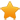Switch Editions?
CancelChannel: CodeSection,代码区,网络安全 - CodeSec
Viewing all articles

# Destoon 6.0 guestbook.php 通用SQL注入漏洞

0
0

mobile/guestbook.php 中将 \$_SERVER['HTTP_USER_AGENT'] 删掉了。分析一下，这里是手机端的留言板，destoon将用户的User-Agent放入了留言内容变量 \$post[content] 中。

function add(\$post) { \$post = \$this->set(\$post); \$sqlk = \$sqlv = ''; foreach(\$post as \$k=>\$v) { if(in_array(\$k, \$this->fields)) { \$sqlk .= ','.\$k; \$sqlv .= ",'\$v'"; } } \$sqlk = substr(\$sqlk, 1); \$sqlv = substr(\$sqlv, 1); \$this->db->query("INSERT INTO {\$this->table} (\$sqlk) VALUES (\$sqlv)"); return \$this->itemid; }

function set(\$post) { global \$DT_TIME, \$_username, \$DT_IP, \$TYPE; \$post['content'] = strip_tags(\$post['content']); \$post['title'] = in_array(\$post['type'], \$TYPE) ? '['.\$post['type'].']' : ''; \$post['title'] .= dsubstr(\$post['content'], 30); \$post['title'] = daddslashes(\$post['title']); \$post['hidden'] = isset(\$post['hidden']) ? 1 : 0; if(\$this->itemid) { \$post['status'] = \$post['status'] == 2 ? 2 : 3; \$post['editor'] = \$_username; \$post['edittime'] = \$DT_TIME; } else { \$post['username'] = \$_username; \$post['addtime'] = \$DT_TIME; \$post['ip'] = \$DT_IP; \$post['edittime'] = 0; \$post['reply'] = ''; \$post['status'] = 2; } \$post = dhtmlspecialchars(\$post); return array_map("trim", \$post); }

content 有如下过程： strip_tags -> htmlspecialchars -> trim title 有如下过程： in_array(\$post['type'], \$TYPE) ? '['.\$post['type'].']' : '' -> substr(\$post['content'], 30) -> addslashes -> trim

==== 分割线 ====

Viewing all articles

More Pages to Explore .....